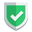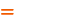我们相信：世界是美好的，你是我也是。平行空间的世界里面，不同版本的生活也在继续...

js和css都被压缩了，那么html有什么理由不被压缩呢？对吧？

## 苏南大叔的答案：

``````\$smarty = new \Smarty;
//......
function compress_html(\$html) {
\$html = preg_replace(':\s+//.*?\n:', '', \$html);
\$html = preg_replace('/<!--\s*[^[][^!][^<].*?-->/s', '', \$html);
\$html = preg_replace('/\/\*.*?\*\//s', '', \$html);
\$html = preg_replace('/>\s*</s', '><', \$html);
\$html = preg_replace('/(\s)+/s', ' ', \$html);
return trim(\$html);
}
\$smarty->registerFilter("output", "compress_html");``````

## 网上流行的说法：

``````function striphtml(\$html)
{
\$html = preg_replace(':\s+//.*?\n:', '', \$html);
\$html = preg_replace('/<!--\s*[^[][^!][^<].*?-->/s', '', \$html);
\$html = preg_replace('/\/\*.*?\*\//s', '', \$html);
\$html = preg_replace('/>\s*</s', '><', \$html);
\$html = preg_replace('/(\s)+/s', ' ', \$html);
return trim(\$html);
}``````

``````public function getContent()
{
return isset(\$this->content) ? \$this->striphtml(\$this->content) : \$this->striphtml(\$this->handler->getContent(\$this));
}``````

## 效果截图如果本文对您有帮助，或者节约了您的时间，欢迎打赏瓶饮料，建立下友谊关系。本博客不欢迎：各种镜像采集行为。请尊重原创文章内容，转载请保留作者链接。【福利】 腾讯云最新爆款活动！1核2G云服务器首年50元！【源码】本文代码片段及相关软件，请点此获取更多信息【绝密】秘籍文章入口，仅传授于有缘之人php    smarty